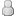• 0 Votes - 0 Average
• 1
• 2
• 3
• 4
• 5
 NPR and IRR
02-02-2009, 02:00 AM
Post: #6
 Muhammad AmirPosting FreakPosts: 782 Joined: Dec 2006 Reputation: 0

Although, the above solution is right but for exam point of view this is laborious. Why don't you use annuity formula?

Present Value Of Annuity = (1/R) â [1/{R*(1+R)^n}].

Where;

R=Interest Rate or Cost of Capital.
n=Number of Periods.

Now, the solution of your question according to annuity formula is.

At Year 21 Annuity Factor is => (1/0.05) â [1/{0.05*(1+0.05)^40}] = 17.159

At Year 18, which is Time 0 Annuity Factor is = > (1/0.05) â [1/{0.05*(1+0.05)^2}] = 1.859

Now The Factor at Year 0 is => 17.159 â 1.859 => 15.3

Present Value of 40 Annuity Receipts is => 15.3 * 5000 => \$76,500

<b>NPV of the project is => \$76,500 â 18,000 => <font size="4">+</font id="size4"> \$58,500</b>

<b>For IRR-</b>

We are already having + NPV of \$58,500 at the discount rate of 5%.

For current pattern of cash flows, discount rate is inversely related to NPV. It means as we increase the interest rate, NPV will be reduced.

If you discount the above cash flows at 25% interest rate you will see a â NPV of -\$5,203.

IRR formula = P rate + [+NPV/+NPV <font size="4"><b>-</b></font id="size4"> (<b>-</b>NPV)] * (P rate â N rate).

Where,

P rate = Rate at +ve NPV
N rate = Rate at âve NPV
+NPV = Positive NPV of Cash flows
-NPV = Negative NPV of Cash flows

IRR = 5% + [58,500/58,500 â (-5,203)]*(25-5) => 23.4%.

<b>IRR = 23.4% at which NPV is Zero.</b>

Now, if you discount your cash flows at 23.4% then you roughly get Zero NPV.
 « Next Oldest | Next Newest »

 Messages In This Thread NPR and IRR - dowerk - 01-29-2009, 02:18 PM [] - kamranACA - 01-29-2009, 08:37 PM [] - dowerk - 01-30-2009, 12:25 AM [] - kamranACA - 01-30-2009, 05:24 PM [] - tariqab - 02-01-2009, 10:45 PM [] - Muhammad Amir - 02-02-2009 02:00 AM [] - kamranACA - 02-02-2009, 06:14 PM [] - tariqab - 02-02-2009, 08:13 PM [] - Muhammad Amir - 02-02-2009, 09:07 PM

Forum Jump:

User(s) browsing this thread: 1 Guest(s)